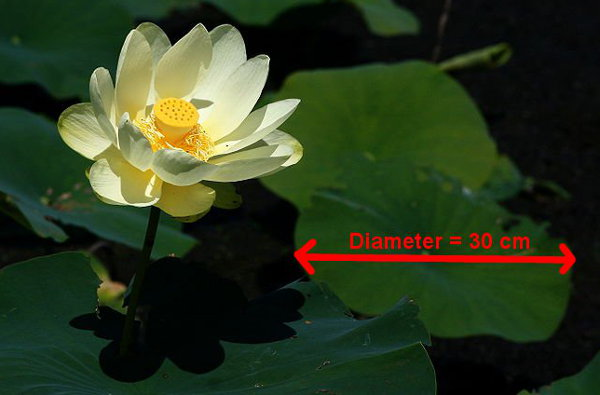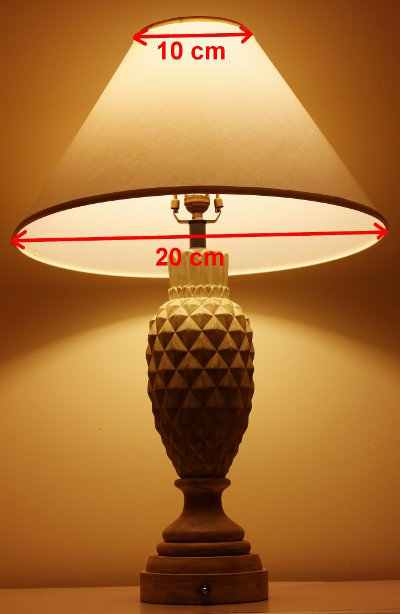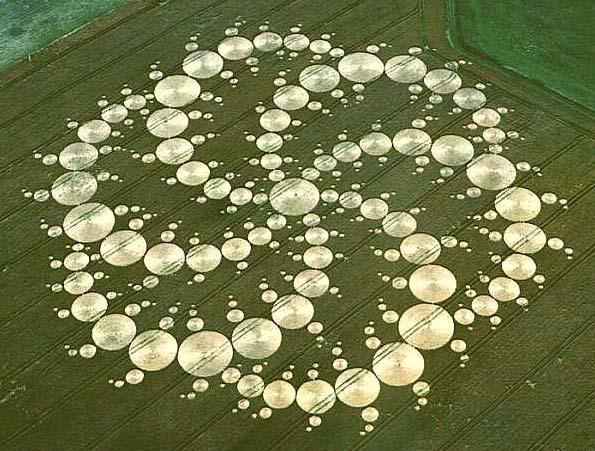# Area Of Circle

The formula is:

Area of Circle = π r2

where
π, pi is a constant, approximately 3.14

Take care to use the Radius and not the Diameter in this rule.

## Example One - Contact Lens SizeA contact lens has a radius of 4 mm. What is its area?
(Assume the surface is flat and that π is 3.14.)

Area of circle
= π r2
= 3.14 × (4 × 4)
= 50.24 mm2

## Example Two - Lotus Lily LeafPlant leaves use sunshine, carbon dioxide and water to photosynthesize and make oxygen and sugar. This lily leaf has a diameter of 30 cm. What is the area to be available for photosynthesis?
(Use π = 3.14.)

Area of circle
= π r2
= 3.14 × (15 × 15)
= 706.5 cm2

## Example Three - Lampshade FabricA lampshade design is made of an annulus with a central angle of 160 degrees. The diameter of the smaller circle is 10 cm. The diameter of the larger circle is 20 cm. What area of fabric is used in the lampshade? (Use π = 3.14.)

Area of larger circle
= π R2
= 3.14 × 10 × 10
= 314 cm2

Area of smaller circle
= π r2
= 3.14 × 5 × 5
= 78.5 cm2

Area of whole annulus
= 314 - 78.5
= 235.5 cm2

Area of 200 degrees of annulus
= (200° ÷ 360°) × 235.5
= 130.8 cm2

## Questions

Find these areas. Use the ∏ button on your calculator.

Q1. A circle whose radius is 30 m
Q2. A circle with a diameter of 40 cm
Q3. A 120 degrees sector whose radius is 9 cm